# OCTAHEDRON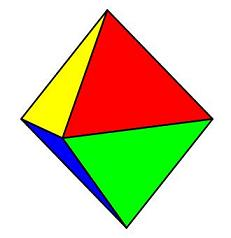See the octahedron rotating around the x, y, z axis.
 Properties of the Octahedron Faces: 8 trianglesVertices: 6, each with 4 edges meeting Edges: 12Dihedral angle: 109°28' The Symmetry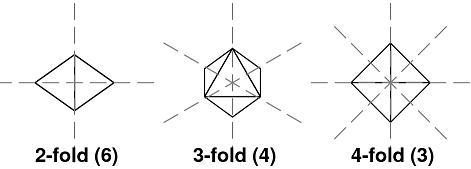Surface Area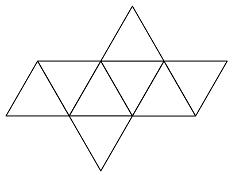Let r = the distance from center to one vertex. The length (a) of edge, by the Pythagoras Theorem, = r√2.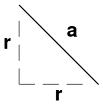Then the area of one triangle is (a × h) / 2, where h = √[a² - (a/2)²]. And the area of the octahedron is 8 × the area of one triangle. Volume The octahedron can be divided into two pyramids.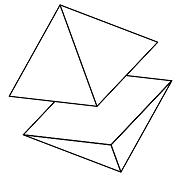The volume of one pyramid = (base area × height) /3. In the case of the regular octahedron, the base area = a². And so, the volume of the octahedron = 2 × the volume of pyramid. V = (√2 / 3)a³